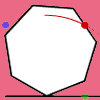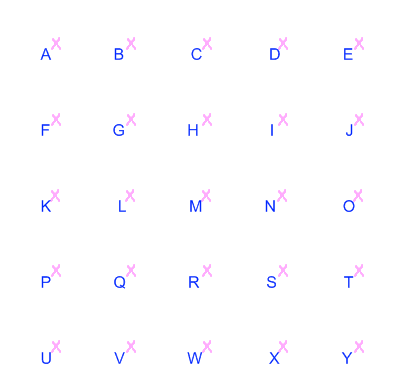#### You may also like### Lying and Cheating

Follow the instructions and you can take a rectangle, cut it into 4 pieces, discard two small triangles, put together the remaining two pieces and end up with a rectangle the same size. Try it!### How Far Does it Move?

Experiment with the interactivity of "rolling" regular polygons, and explore how the different positions of the red dot affects the distance it travels at each stage.### Up and Across

Experiment with the interactivity of "rolling" regular polygons, and explore how the different positions of the dot affects its vertical and horizontal movement at each stage.

# How Steep Is the Slope?

### Why do this problem?

This problem offers an introduction to gradients within a simple environment that allows students to explore all the important aspects of gradient. This is a suitable preparation for students to move on to:

### Possible approach

These printable resources may be useful: How steep is the slope,
Grid Worksheet.

Share with the class the definition of gradient given at the start of the problem. Resist the temptation to give a 'rule' for working out gradients, as methods should emerge in the discussions that follow.

Display a copy of the grid below (a larger version can be found here).Some of the following questions could be used to get students thinking about different gradients:
"I'm picturing a line with a gradient of 2 which goes through H. What other point does it go through?"
"Identify another line with a gradient of 2. How many can you find?"

"Identify a line which is steeper/less steep. Can you find another one?"

"Can you find any lines with gradients between 1 and 2?"

"What is the gradient of the line going through G and O?"

"Can you find a steeper/less steep (negative) line? Can you find another one?"

"Why might it be problematic to work out the gradient of the line going through R and M?"

Use these questions as an opportunity to share different strategies for working out the gradients.

Hand out copies of this sheet and ask students to work in pairs on the main problem:
"How many different gradients can you find? Can you find them all?"
"Arrange them in order of steepness and list the points each line passes through."

Expect students to work systematically so that they are in a good position to justify their findings.
Circulate while students are discussing the problem, and towards the end of the session invite pairs with interesting approaches to share their conclusions with the rest of the group.

Abigail Mantaj from Diocesan School for Girls in Auckland, New Zealand, was inspired by the original problem to create this task for her students.

### Key questions

What does it mean for a line to have positive or negative gradient?

What can be said about lines with positive gradients greater than or less than 1 (or negative gradients greater than or less than -1)?

A standard method for working out gradients is to divide the vertical displacement by the horizontal displacement. What is the connection between this algorithm and the definition of gradient given at the start of the problem?

### Possible support

Students who are struggling to work systematically could be given the prompt, explained in the Hint, to fix one end of their ruler at an extreme point on the grid, and slowly move the other end to create lines with different gradients.

### Possible extension

Students could move on to At Right Angles to consider the gradients of perpendicular lines, or on to Parallel Lines and Perpendicular Lines to investigate the relationship between the equations of parallel and perpendicular lines on a co-ordinate grid.